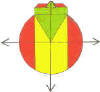DMD   EDU-HOME

WELCOME TO THIS PAGE INTENDED FOR STUDENTS AT THE UNIVERSITY OF THE WESTERN CAPE REGISTERED  FOR THE MODULE:

TUTORIAL TEST 1 (11 Feb)  : SOLUTIONS

1. EIGHT BILLION, ONE MILLION, TWO THOUSAND AND SIXTY FOUR POINT ZERO, ZERO, EIGHT, NINE

Or

EIGHT THOUSAND AND ONE MILLION, TWO THOUSAND AND SIXTY ……ETC.

1. –(14-19) = -(-5) = +5 ( Neg times Neg = Pos)

-12 + 5 - 25 + 21 – 17 :  Sum of  Pos. Numbers = 26

Sum of  Neg. Numbers = 54

Refer to CR, pp.81-82

1. -28 × -4 = 112
2. -13+60 = 47
3. -2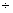(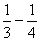)

Is -2()  = -2?

1.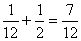2.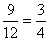3.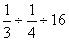=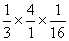=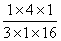=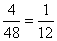Is=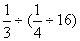?

1.       6
2.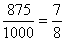3.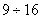= 0.5625 = 56.25%

Use the long or short division ALGORITHM to work this out (or any method which you know of, which does not involve the use of a CALCULATOR)

1.   2 -­­ 600+0.06 = -597.94 metres

NOTE: 1 metre = 0.001 km = 1000 mm = 100 cm

13.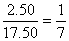14.   2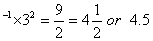15.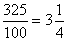16.   R0.26

Use the long MULTIPLICATION ALGORITHM to work this out (or any method which you know of, which does not involve the use of a CALCULATOR)

17.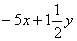18.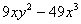19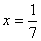20.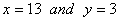©  DESMOND DESAI, DMD EDU-HOME, 2010

All rights reserved. Designed and created by Desmond Desai, South Africa. This page is protected by Copyright. No part of this page may be reproduced, stored in a retrieval system, or transmitted in any form of by any means, electronic, mechanical, photocopying, recording or otherwise, without the prior written permission of the copyright holder.